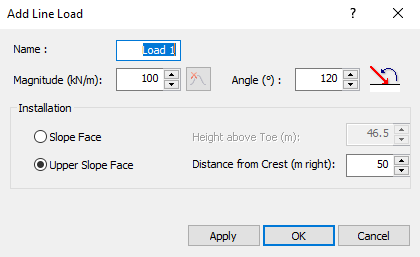Join one of our upcoming courses taking place around the world! Explore now

## Search Results

In RocTopple, a line load is a concentrated load that appears as a point load in the 2D View but that actually represents a linear (line) load when considering the out-of-plane dimension of the model. Hence, the units of a line load are force / length where the length dimension is measured along the slope surface perpendicular to the 2D cross-section. Line loads can be added anywhere along the slope face or upper slope, at any angle, on the exposed upper edges of the toppling blocks.

1. Select Add Line Loadon the toolbar or the Loading menu.2. Enter values for the following parameters:
• Name
• Magnitude
• Angle
• Installation (Slope Face or Upper Slope Face)
A line load appears as an arrow in the 2D View and as a series of arrows joined by a line in the 3D View.

After a load has been added, it can easily be edited, moved or deleted.

The Add Line Load dialog requests the Magnitude as a force per linear distance when considering the out-of-plane dimension of the model. The units correspond to the currently selected Units in the Project Settings dialog.

The Line Load Angle is specified with respect to the horizontal (e.g., a 90 degree load is a vertically oriented load applied downward). Negative angles are permitted.

Line loads can be applied on the slope face or upper slope of a RocTopple model. When you initially add a line load, the installation location is specified as follows:

• Slope Face - The location of the load is specified as the vertical height from the toe of the slope.
• Upper Slope - The location of the load is specified as the horizontal distance from the crest of the slope (even if the upper slope surface is not horizontal).

When a load is added, RocTopple automatically determines the exact location of the load on the toppling block surface corresponding to the number you have entered. If the load does not appear where you intended, you can edit or delete the load and modify your input accordingly.